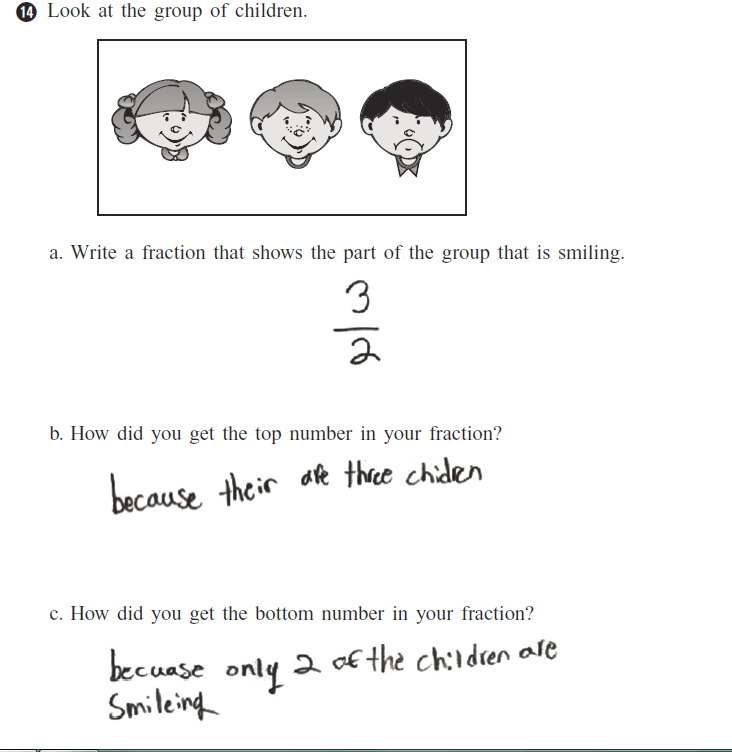Categories

# “3 out of 2 doctors recommend…”What does the student know? What does the student think is going on with fractions? How would you help?

## 4 replies on ““3 out of 2 doctors recommend…””louisesays:

I was going to suggest that the top number is how many are doing the smiling, out of the bottom three available… then realized to my horror that I always say that multiplication means “of ” except for probability when it probably doesn’t. I need a different word.
The student obviously knows how many are available, how many are doing the doing, but is totally at sea about the conventions of fractions/divisions/ratios. Based on prior experience, this student might also put numbers into the calculator backwards (2 then the divide symbol then 3) thereby ending up with the “right answer”. They will also call this “divide two into three”. Undoing this skill is hard when it has been firmly ingrained by 9th grade.
Using manipulatives -? How many pieces in the group? That goes on the bottom. Each piece is less than one whole group. How many of those pieces do you have? The number you have of them multiplies the one on top. If you end up with more than the whole group, something has gone wrong.

The student understands the idea of part to whole, just has it backwards. They can identify the whole and they can identify the part, but they flipped which is the numerator and which is the denominator (and, presumably, what the meaning of the fraction is…).

I suspect if you asked the kid if there were five sets of those exact same three faces (so 15 faces) and asked how many were smiling, you’d get the right answer.Sara Davissays:

There are also developmental considerations. The first thing the child is going to do is look at the picture and see that there are 3 people so that becomes the first numeral in mind, they are then asked how many are smiling. So there might be an issue with how the order of direction is given to where the child wants to write the number. So even if we have taught social/conventional information it may become secondary to what the child SEES.Julie Campossays:

The student knows that there are three children and only two are smiling. They probably are unfamiliar with the significance of a numerator and denominator. In order to help this student I would provide them with the definition of what a numerator is and means and the same for denominator. If this student would have known that the numerator is how many students are smiling, and the denominator is how many children we have in total I think they would have put 2/3. Overall, the student has an idea of fractions but just needs more practice to get the numerator and denominator in the correct spot.